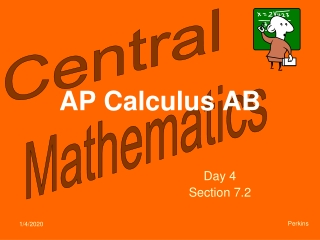DownloadDownload PresentationAP Calculus AB

# AP Calculus AB

Download Presentation## AP Calculus AB

- - - - - - - - - - - - - - - - - - - - - - - - - - - E N D - - - - - - - - - - - - - - - - - - - - - - - - - - -
##### Presentation Transcript

1. AP Calculus AB Day 4 Section 7.2 Perkins

2. How do you find the volume of the figure formed by revolving the shaded area about the x-axis? Outside radius Inside radius The volume we want is the difference between the two. Revolving this function creates a solid whose volume is larger than we want. Revolving this function carves out the part we don’t want.

3. Washer Method (for finding the volume of a solid of revolution) Outside radius Inside radius

4. 1. Find the volume of the solid generated by revolving the area enclosed by the two functions about the x- axis. Find intersection points first. Washer Method:

5. 2. Find the volume of the solid formed by revolving the area enclosed by the given functions about the y- axis. This region is not always created by the same two functions. The change occurs at y = 1. We need to use two integrals to find the volume. For y in [0,1] we use disk method. For y in [1,2] we use washer method. Since we’re revolving about the y-axis, each radius must be in terms of y. (distance from the parabola to the x-axis) (distance from the parabola to the y-axis)

6. AP Calculus AB Day 4 Section 7.2 Perkins

7. How do you find the volume of the figure formed by revolving the shaded area about the x-axis?

8. 1. Find the volume of the solid generated by revolving the area enclosed by the two functions about the x- axis.

9. 2. Find the volume of the solid formed by revolving the area enclosed by the given functions about the y- axis.